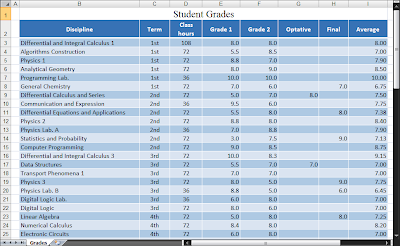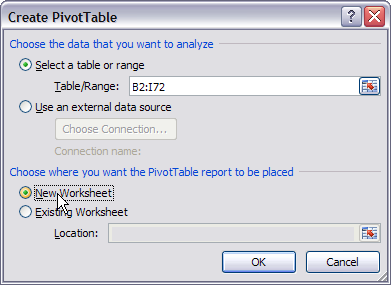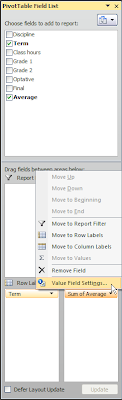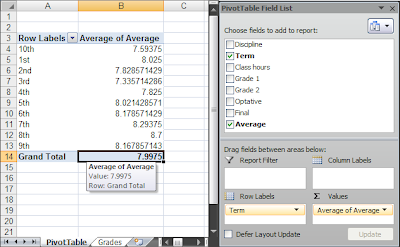## Pages

### PivotTable and PivotChart with Microsoft Excel

In this post I use a sample spreadsheet that stores the grades of a college student to show how to create a pivot table and a pivot chart using Microsoft Excel 2007.

Note: Although I use Microsoft Excel 2007 to illustrate the creation process, I provide at the end of this post a spreadsheet compatible with Microsoft Excel 2003.

Pivot Table
A pivot table is extremely helpful when we want to summarize data through different forms. It gives us the possibility of arranging what data we want summarized and in what shape we want them.

The following is the sample spreadsheet I'm going to use throughout this post:To create a pivot table go to the Insert tab on Microsoft Excel 2007 and select PivotTable.You then can choose where to place the PivotTable. I selected New Worksheet.After doing this, you'll have the following screen:Note that the column headers of the sheet named Grades are included in the PivotTable Field List on the right of the screen.

Just for the sake of organization, let's rename this new sheet PivotTable.

OK. Now we can start summarizing the data as we want. To accomplish this we must choose what fields/columns to add to the report.

What I want to do in this sample case is a simple task: to average the student grades by terms.

I start by selecting the field Term and then I drag and drop it on the the Row Labels area:It's clear that what I'm doing is to represent each term by a row in my report.

Now I'll repeat the drag and drop action but this time with the Average field. I'll put this field on the ∑ Values area.

After doing this last action, we get something like this:At this point we start getting some results; but wait, we're getting the sum of the student's grades for each term. That is not what we want to do. To correct this we must left click the Average field on the ∑ Values area and change its settings:On the new screen that is shown just select Average:Now we have the average grade for each term as we wanted. Look at the final result:The value we get in Grand Total is congruous with the already calculated GPA we have inside the Grades sheet.

Pivot Chart
A pivot chart can be used to display a pivot table's summarized data through different charting types and any changes you make to the pivot table's summarized data will be reflected on the pivot chart.

To create a pivot chart you just have to follow the above steps we used to create a pivot table, but this time instead of selecting a PivotTable you must select PivotChart on the Insert tab.

Bellow is the pivot chart after being formatted:Instead of Row Labels we have Axis Fields (Categories) inside the PivotTable Field List. I rearranged the pivot table report so that the terms are sorted accordingly. I also renamed the fields to reflect more precisely what the report data is about.

Summary
The possibilities for summarizing the data are innumerable and that's what makes a pivot table a so powerful resource.

A pivot chart is simply a chart that uses a pivot table to do data binding.